# Differential operator

A generalization of the concept of a differentiation operator. A differential operator (which is generally discontinuous, unbounded and non-linear on its domain) is an operator defined by some differential expression, and acting on a space of (usually vector-valued) functions (or sections of a differentiable vector bundle) on differentiable manifolds or else on a space dual to a space of this type. A differential expression is a mappingof a setin the space of sections of a vector bundlewith baseinto the space of sections of a vector bundlewith the same base such that for any point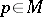and arbitrary sectionsthe coincidence of their-jets (cf. Jet) atentails the coincidence ofand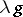at that point. The smallest numberwhich meets this condition for allis said to be the order of the differential expression and the order of the differential operator defined by this expression.

A differential operator on a manifoldwithout boundary often proves to be an extension of an operator which is defined in a natural manner by a fixed differential expression on some set, open in an appropriate topology, of infinitely (or sufficiently often) differentiable sections of a given vector bundlewith base, and thus permits a natural extension to the case of sheaves of germs of sections of differentiable vector bundles. A differential operatoron a manifoldwith boundary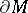is often defined as an extension of an analogous operator which is naturally defined by a differential expression on the set of differentiable functions (or sections of a vector bundle), the restrictions of which tolie in the kernel of some differential operatoron(or satisfies some other conditions definable by some requirements to be satisfied in the domain of values of an operatoron the restrictions of the functions from the domain of definition of, such as inequalities); the differential operatoris said to define the boundary conditions for the differential operator. Linear differential operators on spaces dual to spaces of functions (or sections) are defined as operators dual to the differential operators of the above type on these spaces.

### Examples.

1) Letbe a real-valued function ofvariables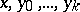, defined in some rectangle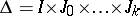; the differential expression(whereusually satisfies some regularity conditions such as measurability, continuity, differentiability, etc.) defines a differential operatoron the manifold, the domain of definitionof which consists of all functionssatisfying the conditionfor. Ifis continuous,may be considered as an operator on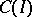with domain of definition; the differential operatoris said to be a general ordinary differential operator. Ifdepends on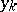, the order ofis.is said to be quasi-linear if it depends linearly on; it is linear ifdepends linearly on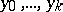; it is said to be linear with constant coefficients ifis independent ofand ifis a linear differential operator. The remaining differential operators are said to be non-linear. If certain conditions as to the regularity ofare satisfied, a quasi-linear operator may be extended to a differential operator from one Sobolev space into another.

2) Let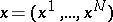run through a domainin, let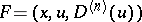be a differential expression defined by a real-valued functionon the product of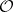and some open rectangle, where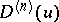is a set of partial derivatives of the type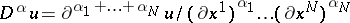, where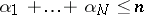, and, as in example 1), let the functionsatisfy certain regularity conditions. The differential operator defined by this expression on the space of sufficiently often differentiable functions onis known as a general partial differential operator. As in example 1), one defines non-linear, quasi-linear and linear partial differential operators and the order of a partial differential operator; a differential operator is said to be elliptic, hyperbolic or parabolic if it is defined by a differential expression of the respective type. One sometimes considers functionsdepending on derivatives of all orders (e.g. as their formal linear combination); such differential expressions, although not defining a differential operator in the ordinary sense, can nevertheless be brought into correspondence with certain operators (e.g. on spaces of germs of analytic functions), and are known as differential operators of infinite order.

3) The previous examples may be extended to include the complex-valued case or the case of functions with values in a locally compact, totally disconnected field and (at least in the case of linear differential operators) even to a more general situation (cf. Differential algebra).

4) Systems of differential expressions define differential operators on spaces of vector functions. For example, the Cauchy–Riemann differential operator, defined by the expression, converts the space of pairs of harmonic functions on the plane into itself.

In the definition of a differential operator and of its generalizations one often employs (besides ordinary derivatives) generalized derivatives, which appear in a natural manner when considering extensions of differential operators defined on differentiable functions, and weak derivatives, related to the transition to the adjoint operator. Moreover, derivatives of fractional and negative orders appear when the differentiation is defined by means of a Fourier transform (or some other integral transform), applicable to the domain of definition and range of such a generalized differential operator (cf. Pseudo-differential operator). This is done in order to obtain the simplest possible representation of the corresponding differential operator of a functionand to attain a reasonable generality in the formulation of problems and satisfactory properties of the objects considered. In this way, a functional or operational calculus is obtained, extending the correspondence between the differentiation operator and the operator of multiplication by the independent variable as realized in the Fourier transform.

Problems in the theory of differential equations — such as problems of existence, uniqueness, regularity, continuous dependence of the solutions on the initial data or on the right-hand side, the explicit form of a solution of a differential equation defined by a given differential expression — are readily interpreted in the theory of operators as problems on the corresponding differential operator defined on suitable function spaces — viz. as problems on kernels, images, the structure of the domain of definition of a given differential operatoror of its extension, continuity of the inverse of the given differential operator and explicit construction of this inverse operator. Problems of the approximation of solutions and of the construction of approximate solutions of differential equations are also readily generalized and improved as problems on the corresponding differential operators, viz. — selection of natural topologies in the domain of definition and in the range such that the operator(if the solutions are unique) realizes a homeomorphism of the domains of definition and ranges in these topologies (this theory is connected with the theory of interpolation and scales (grading) of function spaces, in particular for linear and quasi-linear differential operators). Another example is the selection of differential operators close to a given operator in some definite sense (which makes it possible by using appropriate topologies in the space of differential operators, to justify methods of approximation of equations, such as the regularization and the penalty method, and iterated regularization methods). The theory of differential operators makes it possible to apply classical methods in the theory of operators, e.g. the theory of compact operators, and the method of contraction mappings in various existence and uniqueness theorems for differential equations, in the theory of bifurcation of solutions and in non-linear eigen value problems. Other applications utilize a natural order structure present in function spaces on which a differential operator is defined (in particular, the theory of monotone operators), or use methods of linear analysis (the theory of duality, convex sets, dual or dissipative operators). Again, variational methods and the theory of extremal problems or the presence of certain supplementary structures (e.g. complex, symplectic, etc.) can be used in order to clarify the structure of the kernel and range of the differential operator, i.e. to obtain information on the solution space of the respective equations. Many problems connected with differential expressions necessitate a study of differential inequalities, which are closely connected with multi-valued differential operators.

Thus, the theory of differential operators makes it possible to eliminate a number of difficulties involved in the classical theory of differential equations. The utilization of various extensions of classical differential operators leads to the concept of generalized solutions of the corresponding differential equations (which necessarily proved to be classical in several cases connected with, say, elliptic problems), while the utilization of the linear structure makes it possible to introduce the concept of weak solutions of differential equations. In choosing a suitable extension of a differential operator as defined by a differential expression, a priori estimates of solutions connected with such an expression are of importance, since they permit one to identify function spaces on which the extended operator is continuous or bounded.

Moreover, the theory of differential operators also makes it possible to formulate and solve many new problems, which are qualitatively different from the classical problems in the theory of differential equations. Thus, in the study of non-linear operators it is of interest to study the structure of the set of its stationary points and the action of the operator in a neighbourhood of them, as well as the classification of these singular points, and the stability of the type of the singular point when the respective differential operator is perturbed. Other subjects of interest in the theory of linear differential operators are the description and the study of the spectrum of a differential operator, the calculation of its index, the structure of invariant subspaces of the differential operator, the harmonic analysis of a given differential operator (in particular, the decomposition, which requires a preliminary study of the completeness of the system of eigen functions and associated functions). There is also the study of linear and non-linear perturbations of a given differential operator. These results are of special interest for elliptic differential operators generated by symmetric differential expressions in the context of the theory of self-adjoint operators on a Hilbert space (in particular, in the spectral theory of these operators and the theory of extensions of symmetric operators). The theory of various hyperbolic and parabolic (not necessarily linear) differential operators is connected with the theory of groups and semi-groups of operators on locally convex spaces.

Next to the linear class of differential operators, perhaps the most intensively studied class are differential operators which are either invariant or which vary according to a specific law when certain transformations constituting a group (or a semi-group)are acting in their domain of definition, and hence also on the differential expression. These include, for instance, invariant differential operators connected with the representations of a group; the covariant derivative or, more generally, differential operators on spaces of differentiable tensor fields, whereis the group of all diffeomorphisms (the so-called atomization); many examples of operators in theoretical physics, etc. Such functional-geometric methods are also useful in the study of differential operators with so-called hidden symmetry (see, for example, Korteweg–de Vries equation).

The theory of differential operators as part of the general theory of operators has lately been of increasing importance not merely in the theory of differential equations, but in modern analysis in general. It yields not only important specific examples of unbounded operators (particularly in the theory of linear differential operators), but also tools for the representation and means of study of other objects of various natures. For instance, any generalized function (and even a hyperfunction) is locally obtained by the action of a certain generalized differential operator on a continuous function. Finally, differential operators are becoming more important in other branches of mathematics and effect these to an increasing extend. E.g., one solution of the so-called index problem (cf. Index formulas) connects the topological characteristics of a manifold with the presence of a particular class of differential operators on it; from this it is possible to deduce the properties of elliptic complexes on this manifold.